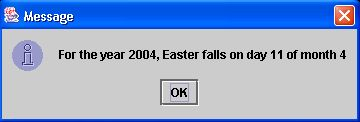### When Is Easter?

8 points

In this program, you calculate the date on which Easter falls for a given year. (Easter falls on the first Sunday after the first full moon in Spring.) A sample run of your program might be like this:
For what year do you want to know the date of Easter? 2001
In 2001, Easter falls on day 15 of month 4.

Quotient and Remainder
Before we get into the program, let's review 2 different ways of handling division. You can divide 2 numbers and keep the quotient (discarding the remainder). For example, when dividing 7 by 2, the quotient is 3 and the remainder is 1. Here is how you would calculate the quotient in Java: quotient = a / b; (where quotient, a and b are integers)

A second way of handling division is to keep the remainder (discarding the quotient). Keeping the remainder is called 'modular arithmetic' or 'mod,' as in '7 mod 2 = 1'. Java uses the '%' symbol for 'mod,' so you might write: remainder = a % b;

It would also be possible to retain both the quotient and the remainder, like this:
quotient = a / b;
remainder = a % b;

calculateEaster()
You will write the method 'calculateEaster()'. The German mathematician Carl Friedrich Gauss in 1800 invented a method to calculate the month and date on which Easter falls.

For this program, all the variables are integers (not doubles). To declare an integer, use the 'int' data type. For example: int squareArea;

Here is his method, which you need to translate into Java code in the method 'calculateEaster()':
1. Let y be the year.
2. Divide y by 19 and call the remainder a. Ignore the quotient.
3. Divide y by 100 to get a quotient b and a remainder c.
4. Divide b by 4 to get a quotient d and a remainder e.
5. Divide (8 * b + 13) by 25 to get a quotient g. Ignore the remainder.
6. Divide (19 * a + b - d - g + 15) by 30 to get a remainder h. Ignore the quotient.
7. Divide c by 4 to get a quotient j and a remainder k.
8. Divide (a + 11 * h) by 319 to get a quotient m. Ignore the remainder.
9. Divide (2 * e + 2 * j - k - h + m + 32) by 7 to get a remainder r. Ignore the quotient.
10. Divide (h - m + r + 90) by 25 to get a quotient n. Ignore the remainder.
11. Divide (h - m + r + n + 19) by 32 to get a remainder p. Ignore the quotient.
12. Easter falls on day p of month n.

After you do the various calculations in 'calculateEaster(),' use 'JOptionPane.showMessageDialog(null, " ")' to print out the month and date of Easter for that year. Here is sample output from my program:main(String[] args)
In 'main(String[] args)'f in 'whenIsEaster.java' (see 'Starting Point' below), you see this line:
input = JOptionPane.showInputDialog("");
This line causes a Dialog box to pop up which the user can use to type in the year. Put the question you are asking the user inside the double-quotes.

year1 = Integer.parseInt(input);
This line converts a String variable (input) to an integer variable (year1).

whenIsEaster easter = new whenIsEaster(year1);
This line creates an object called 'easter' of type 'whenIsEaster' -- the name of the class.

easter.calculateEaster();
This line calls the 'calculateEaster()' method that you are writing.

System.exit(0);
This line is necessary to close the 'thread' that the earlier call to 'JOptionPane.showInputDialog()' opened.

At this website you can check the date that Easter falls.

### Demo

To see how the program runs when it is done, you can download CompletedEaster.jar. Depending on which browser you are using, the program may run if you choose 'Open'. If not, you will have to select 'Save'. After the file downloads, double-click it and the completed program should run. (The file extension may change from .jar to .zip during the download. If this occurs, either while downloading or after downloading rename the file and change the file extension back to .jar.)

### Credit

The idea for this program is taken from Computing Concepts with Java Essentials, 3rd Edition (p. 130), by Cay Horstmann.